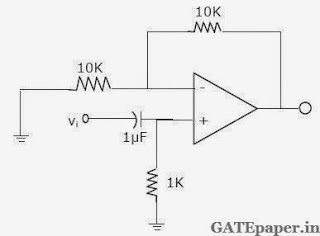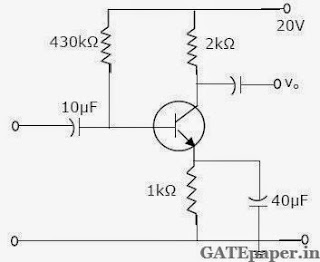### GATE 2005 ECE Video Solutions on Analog Circuits (Analog Electronics)

1. The effect of current shunt feedback in an amplifier is to
a. Increase the input resistance and decrease the output resistance.
b. Increase both input and output resistances
c. Decrease both input and output resistances
d. Decreases the input resistance and increase the output resistance

2. The input resistance Ri of the amplifier shown in figure isa. 30/4 KΩ
b. 10 KΩ
c. 40 KΩ
d. Infinite

3. The cascode amplifier is a multistage configuration of
a. CC – CB
b. CE – CB
c. CB – CC
d. CE – CC

4. A square pulse of 3 volts amplitude is applied to RC circuit shown in figure. The capacitor is initially uncharged. The output voltage Vo at time t = 2 sec isa. 3 volts
b.– 3 volts
c. 4 volts
d. – 4 volts
Solution:

5. For an NPN transistor connected as shown in figure, VBE = 0.7 volts. Given that reverse saturation current of the junction at room temperature 300oK is 10-13 amp, the emitter current isa. 30 mA
b. 39 mA
c. 49 mA
d. 20 mA

6. The voltage eo indicated in figure has been measured by an ideal voltmeter, which of the following can be calculated?a. Bias current of the inverting input only
b. Bias current of the inverting and non-inverting inputs only
c. Input offset current only
d. Both the bias currents and the input offset current.

7. The OP-AMP circuit shown in figure is a filter. The type of filter and its cut-off frequency are respectively.8. In the differential amplifier shown in figure, a large value of REa. Increases both the differential and common mode gains
b. Increases the common mode gain only
c. Decreases the differential mode gain only
d. Decreases the common mode gain only

9. The circuit using a BJT with β = 50 and VBE = 0.7 volts is shown in figure. The base current IB and the collector voltage VC are respectively.a. 43 µA and 11.4 volts
b. 40 µA and 16 volts
c. 45 µA and 11 volts
d. 50 µA and 10 volts

10. Given the ideal operational amplifier circuit shown in figure indicate the correct transfer characteristics assuming ideal diodes with zero cut-in voltage.Common Data Questions for 11, 12 and 13:
Given rd = 20 KΩ, IDSS = 10 mA, VP = - 8 volts.11. Zi and Zo of the circuit are respectively
a. 2 MΩ and 2 KΩ
b. 2 MΩ and 20/11 KΩ
c. Infinity and 2 KΩ
d. Infinity and 20/11 KΩ

12. ID and VDS under DC conditions are respectively
a. 5.625 mA and 8.75 Volts
b. 7.50 mA and 5.0 Volts
c. 4.50 mA and 11.0 Volts
d. 6.25 mA and 7.5 Volts

13. Transconductance in milli-siemens (mS) and the voltage gain of the amplifier are
a. 1.875 mS and 3.41
b. 1.875 mS and – 3.41
c. 3.30 mS and – 6
d. 3.30 mS and 6